# INTRODUCTION TO MATTER MATTER Anything that has mass

• Slides: 25INTRODUCTION TO MATTERMATTER • • Anything that has mass and volume Is everything around you Everything on the Periodic Table All solids, liquids, gases, etc.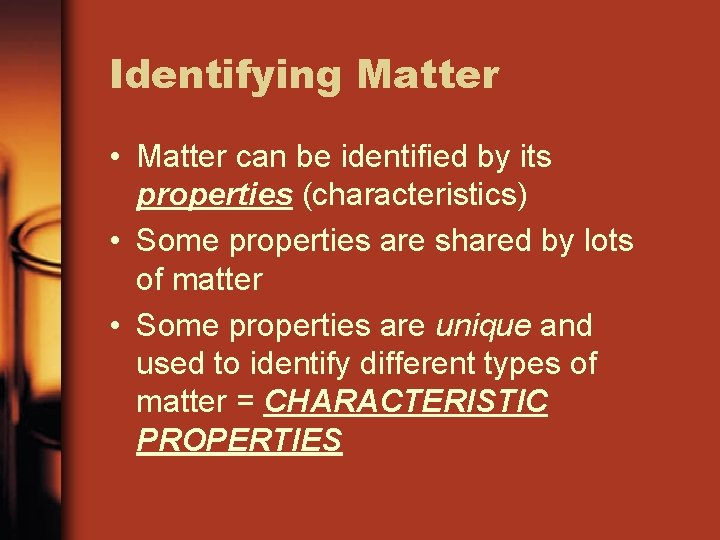Identifying Matter • Matter can be identified by its properties (characteristics) • Some properties are shared by lots of matter • Some properties are unique and used to identify different types of matter = CHARACTERISTIC PROPERTIESPhysical Properties • Physical Properties are properties that you can observe without changing the identity of the substance • Examples: color, shape, size, mass, weight, state, boiling and freezing points, magnetism, tensile strength (stretch), viscosity (flow), malleability (pounded into sheets), density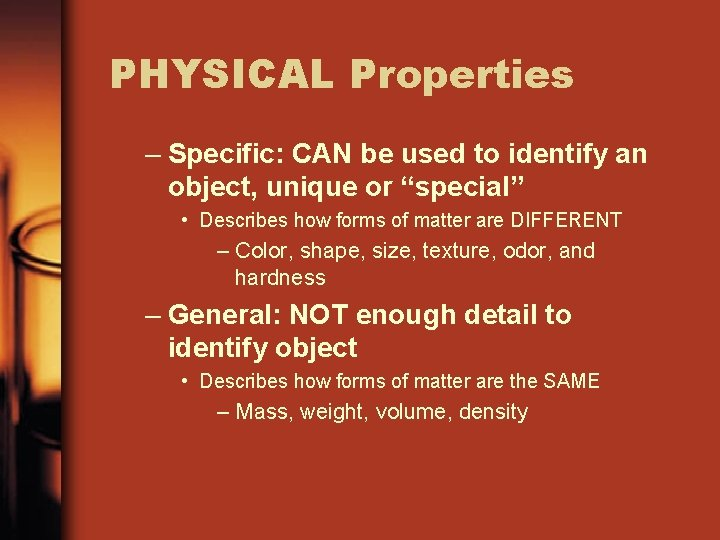PHYSICAL Properties – Specific: CAN be used to identify an object, unique or “special” • Describes how forms of matter are DIFFERENT – Color, shape, size, texture, odor, and hardness – General: NOT enough detail to identify object • Describes how forms of matter are the SAME – Mass, weight, volume, density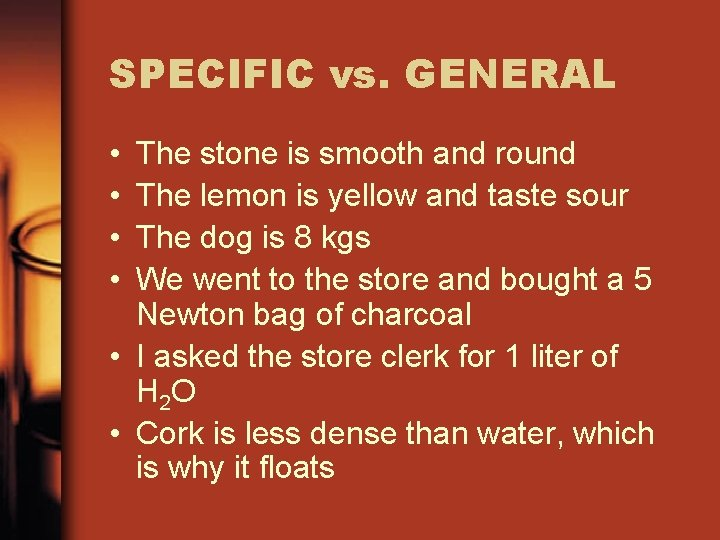SPECIFIC vs. GENERAL • • The stone is smooth and round The lemon is yellow and taste sour The dog is 8 kgs We went to the store and bought a 5 Newton bag of charcoal • I asked the store clerk for 1 liter of H 2 O • Cork is less dense than water, which is why it floatsSPECIFIC vs. GENERAL (cont. ) • Ice is cold and hard • The liquid in the bottle had a strange odor • The square sheet of paper is red on one side and white on the other • The 100 gram piece of metal just balances the rock on the scaleARE DIFFERENT FORMS OF MATTER SIMILAR IN ANY WAY? • Distinguish between matter via properties – Property is a characteristic or description of an object • There are two types of properties/changes – Physical and Chemical – Based on studying chemical reactions and states of matter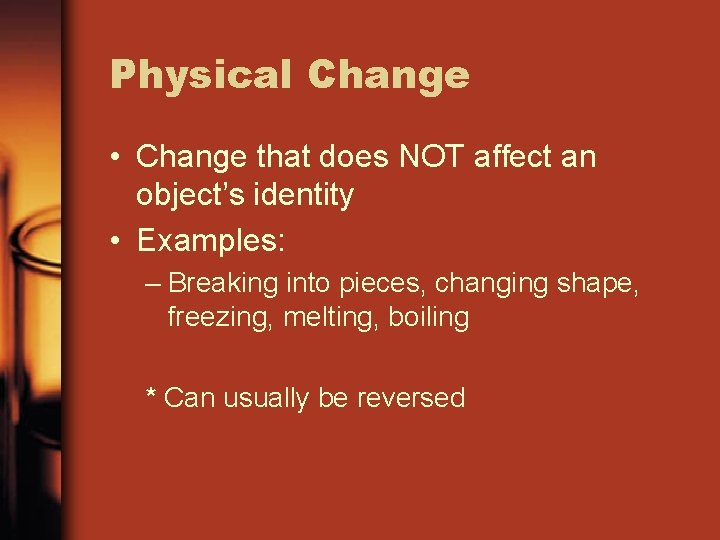Physical Change • Change that does NOT affect an object’s identity • Examples: – Breaking into pieces, changing shape, freezing, melting, boiling * Can usually be reversed• When substances changes states, their identity does not change. As of 1995, 5 states of matter have been identified 1. solid 2. liquid 3. gas 4. plasma 5. Bose-Einstein Condensates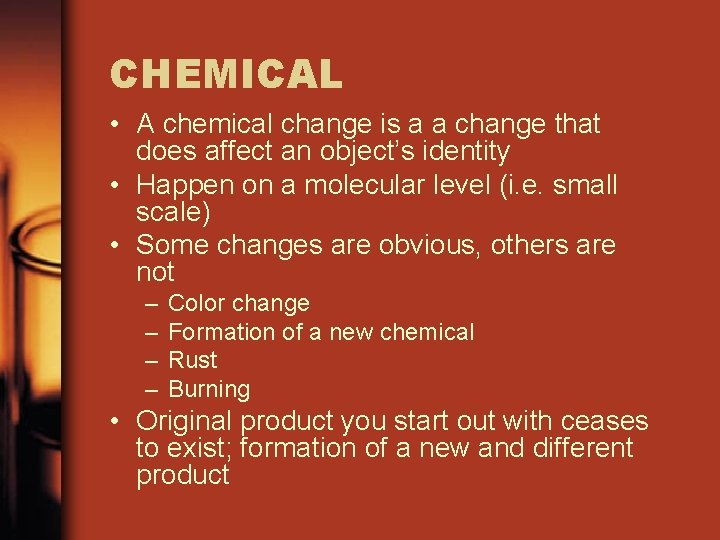CHEMICAL • A chemical change is a a change that does affect an object’s identity • Happen on a molecular level (i. e. small scale) • Some changes are obvious, others are not – – Color change Formation of a new chemical Rust Burning • Original product you start out with ceases to exist; formation of a new and different productChemical Properties • CHEMICAL Properties are properties that indicate whether an object can undergo a chemical change. • Chemical properties cannot be tested with out changing the substance chemically • Examples: – – – Flammable Corrosive Explosive Light sensitive Heat sensitive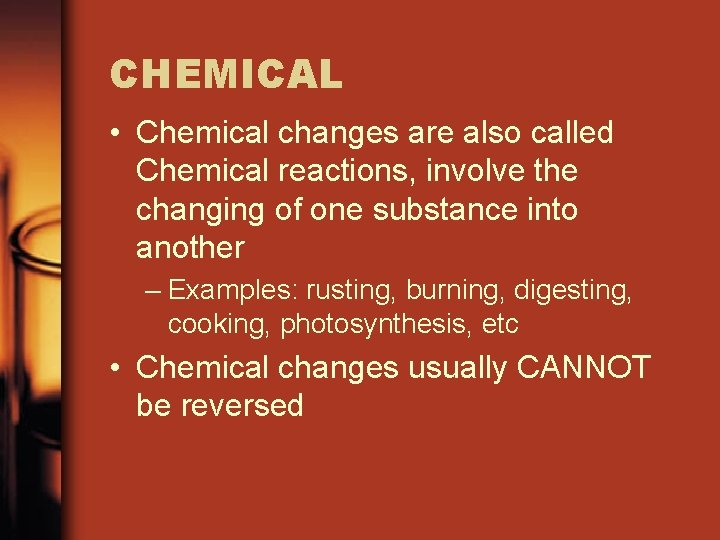CHEMICAL • Chemical changes are also called Chemical reactions, involve the changing of one substance into another – Examples: rusting, burning, digesting, cooking, photosynthesis, etc • Chemical changes usually CANNOT be reversedChemical Changes cont. • How do you know chemical reaction has taken place? 1. 2. 3. 4. • Color change Temperature change Gas produced (bubbles) Precipitate forms When a chemical reaction takes place the mass NEVER changes. The mass of the products always equals the mass of the reactants. – Law of Conservation of Mass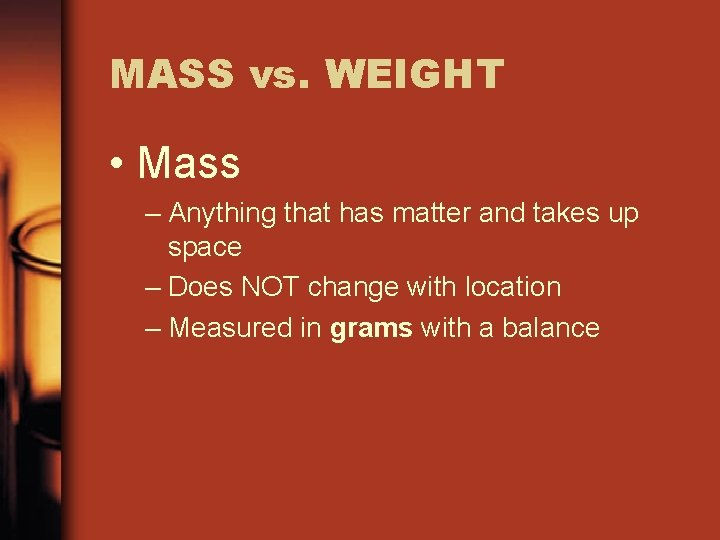MASS vs. WEIGHT • Mass – Anything that has matter and takes up space – Does NOT change with location – Measured in grams with a balance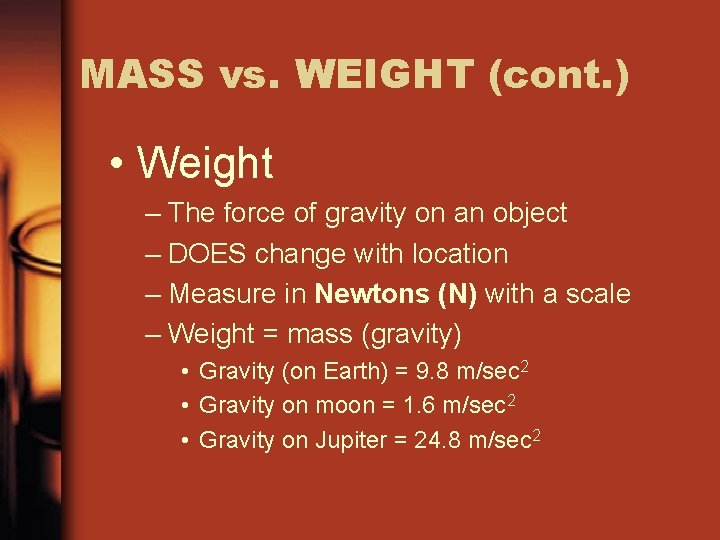MASS vs. WEIGHT (cont. ) • Weight – The force of gravity on an object – DOES change with location – Measure in Newtons (N) with a scale – Weight = mass (gravity) • Gravity (on Earth) = 9. 8 m/sec 2 • Gravity on moon = 1. 6 m/sec 2 • Gravity on Jupiter = 24. 8 m/sec 2MASS vs. WEIGHT (cont. ) • 3 differences between the two – They differ by definition – Weight is a value that changes and depends on location. Mass is a value that remains constant unless matter is added or taken away – They differ in their respective measured units • Weight is measure in Newtons (N) • Mass is measured in grams (g)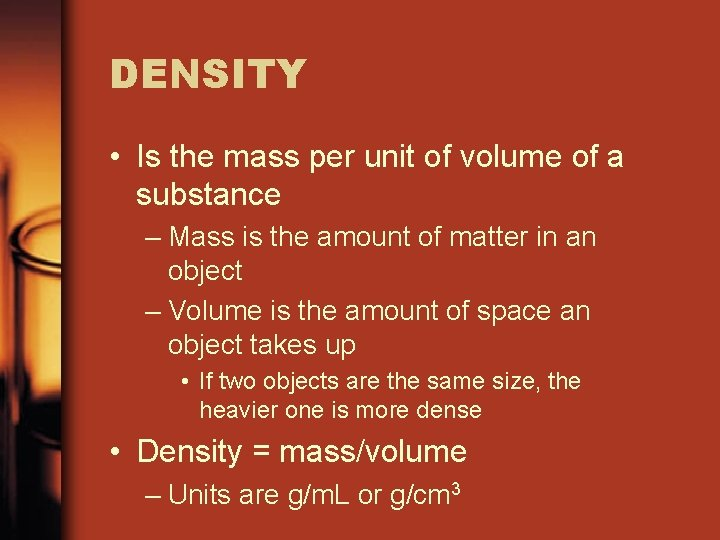DENSITY • Is the mass per unit of volume of a substance – Mass is the amount of matter in an object – Volume is the amount of space an object takes up • If two objects are the same size, the heavier one is more dense • Density = mass/volume – Units are g/m. L or g/cm 3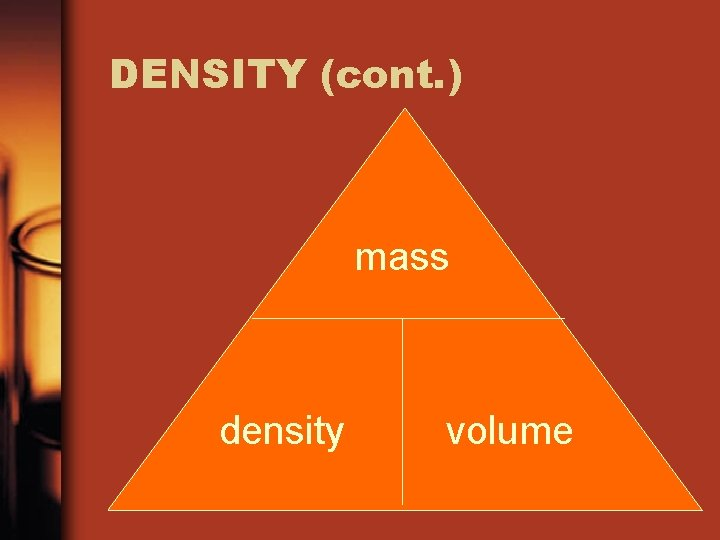DENSITY (cont. ) mass density volume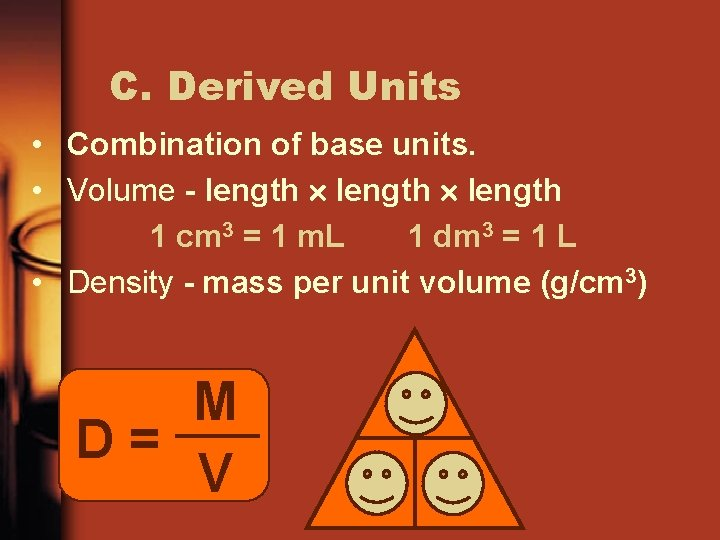C. Derived Units • Combination of base units. • Volume - length 1 cm 3 = 1 m. L 1 dm 3 = 1 L • Density - mass per unit volume (g/cm 3) M D= V M D V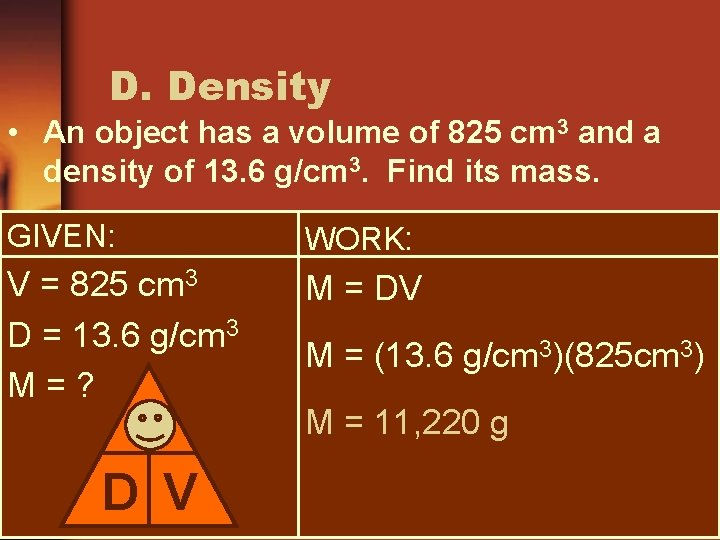D. Density • An object has a volume of 825 cm 3 and a density of 13. 6 g/cm 3. Find its mass. GIVEN: WORK: V = 825 cm 3 D = 13. 6 g/cm 3 M=? M = DV M D V M = (13. 6 g/cm 3)(825 cm 3) M = 11, 220 g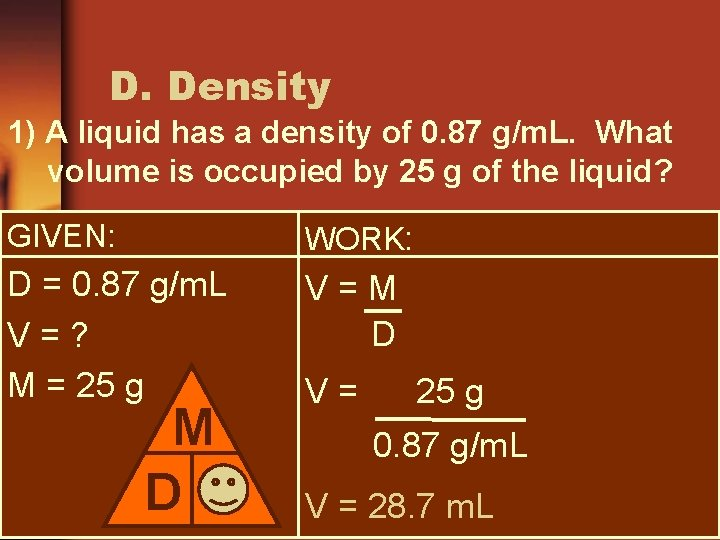D. Density 1) A liquid has a density of 0. 87 g/m. L. What volume is occupied by 25 g of the liquid? GIVEN: WORK: D = 0. 87 g/m. L V=? M = 25 g V=M D V V= 25 g 0. 87 g/m. L V = 28. 7 m. LD. Density 2) You have a sample with a mass of 620 g & a volume of 753 cm 3. Find density. GIVEN: WORK: M = 620 g V = 753 cm 3 D=? D=M V M D V D= 620 g 753 cm 3 D = 0. 82 g/cm 3DENSITY (cont. ) • Density of an object will determine is the object will float or sink • If an object floats in a fluid, the density of that object is less than the fluid’s density • If an object sinks in a fluid, the density of that object is greater than the fluid’s density • Density of water is 1. 0 g/m. LDENSITY (cont. ) • An unknown substance has a mass of 36. 4 g and a volume of 10 m. L. What is the density? • A substances has a mass of 62. 55 g and a volume of 4. 8 cm 3. What is the density of the substance? • A piece of steel has a mass of 35 g and a density of 7. 8 g/cm 3. What is the volume of the steel? • A substance has a density of 19. 3 g/m. L and a volume of 2. 45 m. L. What is the mass of the substance?